# RD Sharma Class 8 Solutions Chapter 13 Profits, Loss, Discount and Value Added Tax (VAT) Ex 13.1

In this chapter, we provide RD Sharma Class 8 Solutions Chapter 13 Profits, Loss, Discount and Value Added Tax (VAT) Ex 13.1 for English medium students, Which will very helpful for every student in their exams. Students can download the latest RD Sharma Class 8 Solutions Chapter 13 Profits, Loss, Discount and Value Added Tax (VAT) Ex 13.1 Maths pdf, free RD Sharma Class 8 Solutions Chapter 13 Profits, Loss, Discount and Value Added Tax (VAT) Ex 13.1 book pdf download. Now you will get step by step solution to each question.

### RD Sharma Solutions for Class 8 Chapter 13 Profits, Loss, Discount and Value Added Tax (VAT) Ex 13.1Download PDF

Question 1.
A student buys a pen for Rs. 90 and sells it for Rs. 100. Find his gain and gain percent ?
Solution:
C.P. of a pen = Rs. 90
and S.P. = Rs. 100
Gain = S.P. – C.P. = Rs. 100 – 90 (S.P > C.P.)
= Rs. 10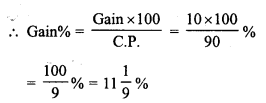Question 2.
Rekha bought a saree for Rs. 1240 and sold it for Rs. 1147. Find her loss and loss percent.
Solution:
C.P. of saree.= Rs. 1240 and
S.P. = Rs. 1147
Loss = C.P – S.P. = Rs. 1240 – Rs. 1147 (C.P. > S.P.)
= Rs. 93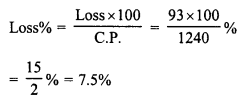Question 3.
A boy buys 9 apples for Rs. 9.60 and sells them at 11 for Rs. 12. Find his gain or loss percent.
Solution:
L.C.M. of 9 and 11 = 99
Let 99 apples were purchased.
C.P. of 99 apples at the rate of 9 apples for Rs. 9.60Question 4.
The cost price of 10 articles is equal to the selling price of 9 articles. Find the profit percent.
Solution:
C.P. of 10 articles = S.P. of 9 articles = 90 (Suppose)Question 5.
A retailer buys a radio for Rs. 225. His overhead expenses are Rs. 15. If he sells the radio for Rs. 300, determine his profit percent.
Solution:
Cost of radio = Rs. 225
Over head expenses = Rs. 15
Total C.P. of the ratio = Rs. 225 + 15 = Rs. 240
S.P. of radio = Rs. 300
Gain = S.P. – C.P. = Rs. 300 – Rs. 240 = Rs. 60Question 6.
A retailer buys a cooler for Rs. 1200 and overhead expenses on it are Rs. 40. If he sells the cooler for Rs. 1550, determine his profit percent.
Solution:
Cost of cooler = Rs. 1200
Total cost price of cooler = Rs. 1200 + Rs. 40 = Rs. 1240
Selling price = Rs. 1550
Gain = S.P. – C.P. = Rs. 1550 – Rs. 1240 = Rs. 310Question 7.
A dealer buys a wristwatch for Rs. 225 and spends Rs. 15 on its repairs. If he sells the same for Rs. 300, find his profit percent.
Solution:
Cost of wristwatch = Rs. 225
Cost on repairs = Rs. 15
Total cost price = Rs. 225 + Rs. 15 = Rs. 240
Selling price = Rs. 300
Gain = S.P. – C.P. = Rs. 300 – Rs. 240 = Rs. 60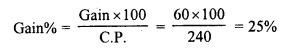Question 8.
Ramesh bought two boxes for Rs. 1300. He sold one box at a profit of 20% and the other box at a loss of 12%. If the selling price of both boxes is the same, find the cost price of each box.
Solution:
Total cost price of two boxes = Rs. 1300
S.P. of each box is same.
Let S.P of each box = Rs. 100
S.P. of first box = Rs. 100
Gain = 20%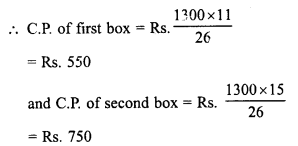Question 9.
If the selling price of 10 pens is equal to cost price of 14 pens, find the gain percent.
Solution:
S.P. of 10 pens = C.P. of 14 pens = Rs. 100 (Suppose)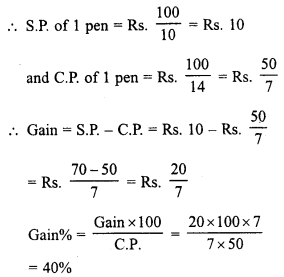Question 10.
If the cost price of 18 chairs be equal to selling price of 16 chairs, And the gain or loss percent.
Solution:
C.P. of 18 chairs = S.P. of 16 chairs = Rs. 100 (Suppose)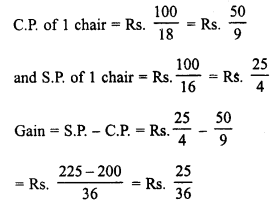Question 11.
If the selling price of 18 oranges is equal to the cost price of 16 oranges, And the loss percent.
Solution:
S.P. of 18 oranges = C.P. of 16 oranges = Rs. 100 (Suppose)Question 12.
Ravish sold his motorcycle to Vineet at a loss of 28%. Vineet spent Rs. 1680 on its repairs and sold the motor cycle to Rahul for Rs. 35910, thereby making a profit of 12.5%, find the cost price of the motor cycle for Ravish.
Solution:
Cost price of cycle for Rahul or Selling price for Vineet = Rs. 35910
Gain = 12.5%Question 13.
By selling a book for Rs. 258, a book-seller gains 20%. For how much should he sell it to gain 30% ?
Solution:
S.P. of a book = Rs. 258
Gain = 20%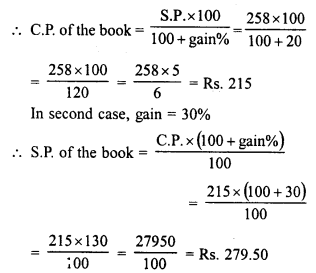Question 14.
A defective briefcase costing Rs. 800 is being sold at a loss of 8%. If its price is further reduced by 5%, find its selling price.
Solution:
C.P. of a briefcase = Rs. 800
Loss = 8%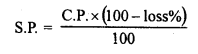Question 15.
By selling 90 ball pens for Rs. 160, a person losses 20%. How many ball pens should be sold for Rs. 96 so as to have a profit of 20%.
Solution:
S.P of 90 ball pens = Rs. 160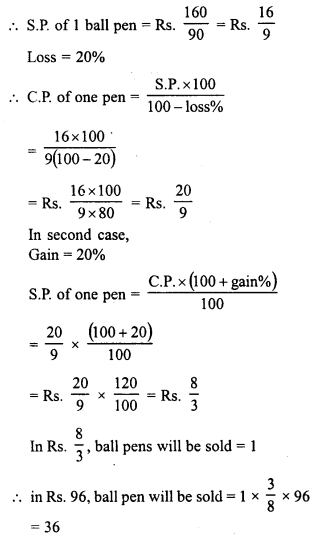Question 16.
A man sells an article at a profit of 25%. If he had bought it at 20% less and sold it for Rs. 36.75 less, he would have gained 30%. Find the cost price of the article.
Solution:
Let C.P. of the article = Rs. 100
In first case gain = 25%
S.P. = 100 + 25 = Rs. 125
In second case,
C.P. = 20% less of Rs. 100 = 100 – 20 = Rs. 80
Gain = 30%Question 17.
A dishonest shopkeeper professes to sell pulses at his cost price but uses a false weight of 950 for each kilogram. Find his gain percent.
Solution:
Let C.P. of 1 kg of pulses = Rs. 100
S.P. of 950 gm = Rs. 100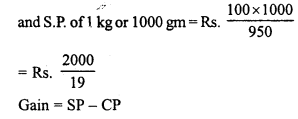Question 18.
A dealer bought two tables for Rs. 3120. He sold one of them at a loss of 15% and other at a gain of 36%. Then he found that each table was sold for the same price, find the cost price of each table.
Solution:
Cost price of two tables = Rs. 3120
Let S.P of each table = Rs. 100
Now S.P of one table = Rs. 100
Loss = 15%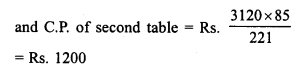Question 19.
Mariam bought two fans for Rs. 3605. She sold one at a profit of 15% and the other at a loss of 9%. If Mariam obtained the same amount for each fan, find the cost price of each fan.
Solution:
Total cost price of two fans = Rs. 3605
Let selling price of each fan = Rs. 100
Now S.P. of first fan = Rs. 100
Profit = 15%Question 20.
Some toffees are bought at the rate of 11 for Rs. 10 and the same number at the rate of 9 for Rs. 10. If the whole lot is sold at one rupee per toffee, find the gain or loss percent on the whole transaction.
Solution:
L.C.M. of 11 and 9 = 99
Let each time 99 toffees are bought.
In first case, the C.P. of 99 toffees at the rate of 11 for Rs. 10Question 21.
A tricycle is sold at a gain of 16%. Had it been sold for Rs. 100 more, the gain would have been 20%. Find the C.P. of the tricycle.
Solution:
Let the C.P. of tricycle = Rs. 100
In first case, gain = 16%
S.P. = Rs. 100 + 16 = Rs. 116
In second case, gain = 20%
S.P. = Rs. 100 + 20 = Rs. 120
Difference in S.P.’s = Rs. 120 – Rs. 116 = Rs. 4
If difference is Rs. 4,
then C.P. of the tricycle = Rs. 100
and if difference is Re. 1, then C.P.Question 22.
Shabana bought 16 dozen ball pens and sold then at a loss equal to S.P. of 8 ball pens. Find
(i) her loss percent
(ii) S.P. of 1 dozen ball pens, if she purchased these 16 dozen ball pens for Rs. 576.
Solution:
C.P. of 16 dozen ball pens = S.P. of 16 dozen pens – loss
C.P. of 16 x 12 pens = S.P. of 16 dozen pens x S.P. of 8 pens
C.P. of 192 pens = S.P. of 16 x 12 pens x S.P. of 8 pens
S.P. of 192 pens + S.P. of 8 pens = S.P. of 200 pens
(i) Let C.P. of 1 pen = Re. 1
Then C.P. of 192 pens = Rs. 192
and S.P. of 200 pens = Rs. 192Question 23.
The difference between two selling prices of a shirt at profits of 4% and 5% is Rs. 6. Find
(i) C.P. of the shirt
(ii) The two selling prices of the shirt
Solution:
The difference of two selling prices of shirt = Rs. 6
Difference in profits of 4% and 5% = 5 – 4 = 1%
(i) C.P. = 1 x 6 x 100 = Rs. 600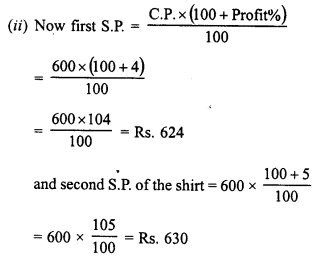Question 24.
Toshiba bought 100 hens for Rs. 8000 and sold 20 of these at a gain of 5%. At what gain percent she must sell the remaining hens so as to gain 20% on the whole ?
Solution:
Total number of hens bought = 100
C.P. of 100 hens = Rs. 8000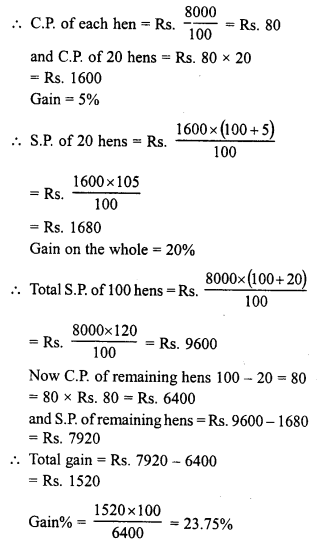All Chapter RD Sharma Solutions For Class 8 Maths

—————————————————————————–

All Subject NCERT Exemplar Problems Solutions For Class 8

All Subject NCERT Solutions For Class 8

*************************************************

I think you got complete solutions for this chapter. If You have any queries regarding this chapter, please comment on the below section our subject teacher will answer you. We tried our best to give complete solutions so you got good marks in your exam.

If these solutions have helped you, you can also share rdsharmasolutions.in to your friends.